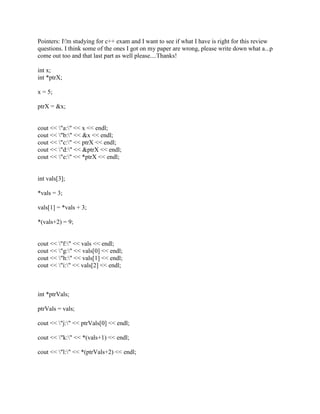Publicité

# Pointers- I-'m studying for c++ exam and I want to see if what I have.docx

rtodd884
8 Feb 2023Prochain SlideShare901131 examples
Chargement dans ... 3
1 sur 3
Publicité

### Pointers- I-'m studying for c++ exam and I want to see if what I have.docx

1. Pointers: I'm studying for c++ exam and I want to see if what I have is right for this review questions. I think some of the ones I got on my paper are wrong, please write down what a...p come out too and that last part as well please....Thanks! int x; int *ptrX; x = 5; ptrX = &x; cout << "a:" << x << endl; cout << "b:" << &x << endl; cout << "c:" << ptrX << endl; cout << "d:" << &ptrX << endl; cout << "e:" << *ptrX << endl; int vals; *vals = 3; vals = *vals + 3; *(vals+2) = 9; cout << "f:" << vals << endl; cout << "g:" << vals << endl; cout << "h:" << vals << endl; cout << "i:" << vals << endl; int *ptrVals; ptrVals = vals; cout << "j:" << ptrVals << endl; cout << "k:" << *(vals+1) << endl; cout << "l:" << *(ptrVals+2) << endl;
2. int *ptr = NULL; cout << "m:" << ptr << endl; const int intRate = 55; const int *ptrRate; ptrRate = &intRate; cout << "n:" << intRate << endl; *ptrRate = 66; cout << "o:" << intRate << endl; int * const ptrY = &x; ptrY = &vals; cout << "p:" << *ptrY << endl; const int * const ptrZ = &intRate; ptrZ = &vals; *ptrZ = 7; Solution a)5 b)b=address of location where x istored. c)address of value x
3. d)address of value x stored in address of ptrX e)5 f)3 g)3 h)6 i)9
Publicité